# Subquery Diagramming

## Simple join and non-correlated subqueries

Simple join

select * from a, b where b.f=a.f
Non-correlated subquery is basically the same

select * from a,
(select b.f1 from b where b.f2=10)
c
where c.f1=a.f1
What about aggregate non-correlated sub-queries/ They aren't exactly the same since they are more difficult rewrite as a regular join:
select ename from emp, (select deptno from emp group by deptno having count(*) > 1) a where a.deptno=emp.deptno;

## Single row subquery

Special case, where non correlated subquery only returns one row

select * from a where a.f1 =
(select max(b.f1) from b )
(laid out side by side because it acts as a filter not a master detail relationship)

## Correlated subquery

select * from a where a.sal >
(select avg(sal) from b where b.f2=a.f2)
Alternatively an arrow could be included to indicate that the subquery could be run for every row returned in the out query
Is there a need to distinguish between aggregated correlated sub-queries and just correlates sub-queries.

### Example

SELECT
A.COMPANY
, A.PAYGROUP
, E.OFF_CYCLE
, E.SEPCHK_FLAG
, E.TAX_METHOD
, E.TAX_PERIODS
, C.RETROPAY_ERNCD
, sum(C.AMOUNT_DIFF) SUM_AMOUNT
from PS_PAY_CALENDAR A
, WB_JOB B
, WB_RETROPAY_EARNS C
, PS_RETROPAY_RQST D
, PS_RETROPAYPGM_TBL E
where A.RUN_ID = 'PD2'
and A.PAY_CONFIRM_RUN = 'N'
and B.COMPANY = A.COMPANY
and B.PAYGROUP = A.PAYGROUP
and E.OFF_CYCLE = A.PAY_OFF_CYCLE_CAL
and B.EFFDT = (SELECT
/*+ qb_name(wb_hj) */
MAX(F.EFFDT)
from WB_JOB F
where F.EMPLID =B.EMPLID
and F.EMPL_RCD# = B.EMPL_RCD#
and F.EFFDT< = A.PAY_END_DT)
nd B.EFFSEQ = a(SELECT MAX(G.EFFSEQ)
from WB_JOB G
where G.EMPLID = B.EMPLID
and G.EMPL_RCD# = B.EMPL_RCD#
and G.EFFDT = B.EFFDT)
and C.EMPLID = B.EMPLID
and C.EMPL_RCD# = B.EMPL_RCD#
and C.RETROPAY_PRCS_FLAG = 'C'
and D.RETROPAY_SEQ_NO = C.RETROPAY_SEQ_NO
and E.RETROPAY_PGM_ID = D.RETROPAY_PGM_ID
group by A.COMPANY
, A.PAYGROUP
, E.OFF_CYCLE
, E.SEPCHK_FLAG
, E.TAX_METHOD
, E.TAX_PERIODS
, C.RETROPAY_ERNCD
/
I originally diagram this as
but the subqeries are really only on G and F and the correlate with tables in the outside query:

## Outer Joins

If English and French both have a unique key on the "ordinal_id" then it's basically one-to-one relationship
We add an arrow in the middle of the line to denote "outer join". The arrow points from the table that drives the join, ie all the rows in the table pointed from are returned even if a match isn't found in the table pointed to.
above graphic originally on http://blog.mclaughlinsoftware.com/oracle-sql-programming/basic-sql-join-semantics/

 type ANSI ANSI 89 (Oracle) type type inner join english INNER JOIN french using (ordinal_id) english e, french f where e.ordinal_id=f.ordinal_id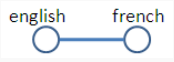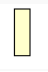left outer join english LEFT JOIN french using (ordinal_id) english e, french f where e.ordinal_id=f.ordinal_id(+)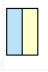right outer join english RIGHT JOIN french using (ordinal_id) english e, french f where e.ordinal_id(+)=f.ordinal_id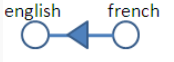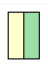full join english FULL JOIN french using (ordinal_id) english e, french f where e.ordinal_id=f.ordinal_id(+) UNION english e, french f where e.ordinal_id(+)=f.ordinal_id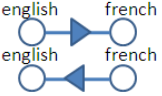## Semi Joins

SELECT *
FROM dept d WHERE exists ( SELECT null FROM emp e WHERE e.deptno=d.deptno);
SELECT *
FROM dept d WHERE d.deptno in (SELECT deptno FROM emp e );

## Not/Not Exists

SELECT *
FROM dept d WHERE not exists ( SELECT null FROM emp e WHERE e.deptno=d.deptno);
SELECT *
FROM dept d WHERE d.deptno not in ( SELECT deptno FROM emp e );